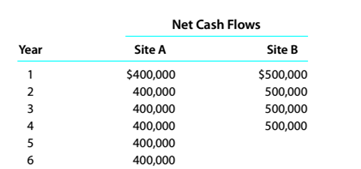Chapter 15, Problem 15.5.2P

Chapter
Section
Textbook Problem

Evaluate alternative capital investment decisionsThe investment committee of Iron Skillet Restaurants Inc. is evaluating two restaurant sites.The sites have different useful lives, hut each requires an investment of $1.000,000. Theestimated net cash flows from each site are as follows:The committee has selected a rate of 20% for purposes of net present value analysis.It also estimates that the residual value at the end of each restaurant’s useful life is$0,but at the end of the fourth year. Site A’s residual value would be $300.000. InstructionsFor each site, compute the net present value, assuming that Site A is adjusted to afour-year life for purposes of analysis. Use the present value of$1 table appearing inthis chapter.

To determine

Concept Introduction:

NPV:

Net present value (NPV) is the method to evaluate the project feasibility. This method calculates the present value of cash inflows and outflows, and then calculates the net present value of the investment. A project should be accepted if it has a positive NPV. The formula to calculate the NPV is as follows:

NPV = Present value of cash inflows  Present value of cash out flows

To Calculate:

The Net present value for each site

Explanation

The Net present value for each site is calculated as follows:

 Site A Net Cash Flows PVF (20%) PV A B =A*B Year 0 $(1,000,000) 1.0000$ (1,000,000) Year 1 $400,000 0.8333$ 333,333 Year 2 $400,000 0.6944$ 277,778 Year 3 $400,000 0.5787$ 231,481 Year 4 \$ 700,000 0...

Still sussing out bartleby?

Check out a sample textbook solution.

See a sample solution

The Solution to Your Study Problems

Bartleby provides explanations to thousands of textbook problems written by our experts, many with advanced degrees!

Get Started

Find more solutions based on key concepts